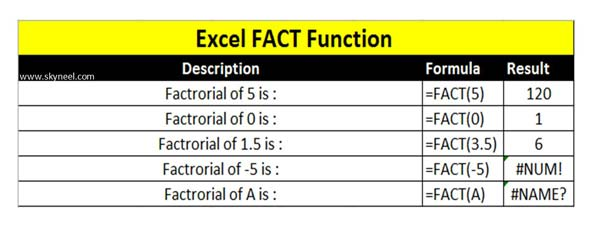# Excel Fact Function – Return Factorial of Number

Excel Fact Function located in Math & Trig category which allows you to returns the factorial of any positive number in active worksheet.

Excel Fact function is used to returns the factorial of given positive number. The Fact function in Excel is located in the Math & Trig category. The factorial of a positive number is equal to 1*2*3*…. number. If you need to quickly calculate factorial of any large number then you can use Excel Fact function in active worksheet. In this article we have to describe the formula and how to use Excel Fact function.

The Microsoft Excel Fact function allows you to quickly returns the factorial of any positive number. Fact is an important built-in function which is located in the Math & Trig category. This function can be used just like a worksheet (WS) function. You can use Fact function to get the factorial either directly fill the number or use cell address in active worksheet.

Syntax of Excel Fact Function

FACT(number)

The FACT function has only number arguments:

Number: Required. You have to give any no-negative number for which you want the factorial. If given number is not an integer then, decimal portion has been truncated.

If you try to calculate factorial of any negative number then you will get #NUM! error message in active cell. Just like this you can also get #NAME? error message when you try to calculate factorial of any non-numeric value in active cell. So, you must always remember fact function return the factorial only a positive number. If you have any number with decimal value then decimal places are removed and factorial calculated only on non-decimal value.

## How to use Excel Fact Function

If you want to use Fact function in your active worksheet then you have to check this.• If you want to calculate factorial of any positive number like 5 then you have to type the given function in any cell: =FACT(5) and press enter you will get 120 as result.
• Most of the person want to know the factorial of 0 number then you have to use given function: =FACT(0) and press enter you will get 1 as a result.
• If you try to calculate factorial of any negative number or non-numeric value then you will get an error message in active cell.
• =FACT(-5) returns #NUM! error value.
• =FACT(A) returns #NAME? error value.

Must Read: How to use Excel RAND Function

Always remember if you want to become an Excel expert then you must have better knowledge of Excel functions. I hope after reading this guide you can easily use Fact function. If you have any query or suggestion then send us via comment box.